# Geometry, Student Edition: Geometry Chapter 10 Review Flashcards

Set Details Share
created 9 years ago by kstedman
881 views
This card set includes all of the formulas needed for the Ch. 10 Test
Subjects:
geometry, circles, mathematics
Page to share:
Embed this setcancel
COPY
code changes based on your size selection
Size:
X

1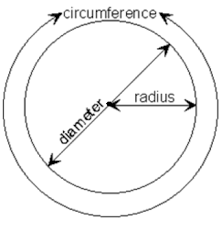What is the circumference of this circle equal to?

C = 2(pi)r = (pi)d

2What is the area of this circle?

A = (pi) r2

3Find the area of this circle.

A = (pi)*36

4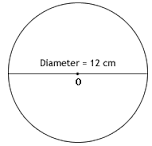Find the circumference of this circle.

C = 12(pi)

5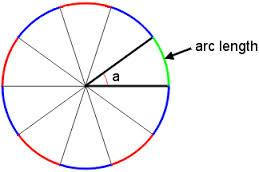What is the arc measure, in degrees?

ao

6What is the arc length?

arc length = a/360 (2)(pi)r = a/360 (pi)d

7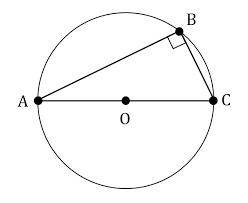If AB = 12 and BC = 5, what equation can you use to find the diameter, d?

52 + 122 = d2

8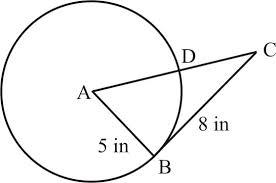What is the correct equation to find x, if x is the length of AC?

52 + 82 = x2

9What is the arc measure of the intercepted arc?

2xo

10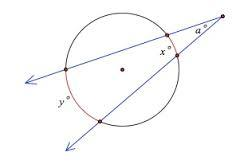What is the measure of <a?

a = 1/2 (y - x)

11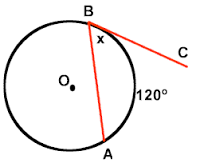Find x.

x = 60o

12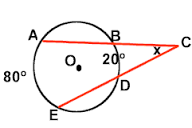Find x.

x = 1/2 (80 - 20) = 1/2 (60) = 30

13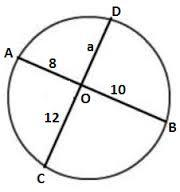What is the correct equation to solve for a?

12a = 8(10)

14What is the correct equation to solve for x ?

18(x + 18) = 16(16 + 24)

15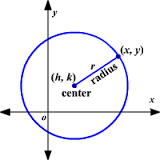What is the equation for this circle?

(x - h)2 + (y - k)2 = r2

16What is the equation for this circle?

(x - 4)2 + (y + 2)2 = 16